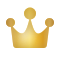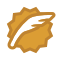# Python 数据分析三剑客之 Matplotlib（八）：等高线 / 等值线图的绘制

CSDN 课程推荐：《Python 数据分析与挖掘》，讲师刘顺祥，浙江工商大学统计学硕士，数据分析师，曾担任唯品会大数据部担任数据分析师一职，负责支付环节的数据分析业务。曾与联想、亨氏、网鱼网咖等企业合作多个企业级项目。

Matplotlib 系列文章：

这里是一段防爬虫文本，请读者忽略。



## 【1x00】等高线概念

• 位于同一等高线上的地面点，海拔高度相同。但海拔高度相同的点不一定位于同一条等高线上；
• 在同一幅图内，除了陡崖以外，不同高程的等高线不能相交；
• 在图廓内相邻等高线的高差一般是相同的，因此地面坡度与等高线之间的等高线平距成反比，等高线平距愈小，等高线排列越密，说明地面坡度越大；等高线平距愈大，等高线排列越稀，则说明地面坡度愈小；
• 等高线是一条闭合的曲线，如果不能在同一幅内闭合，则必在相邻或者其他图幅内闭合。
• 等高线经过山脊或山谷时改变方向，因此，山脊线或者山谷线应垂直于等高线转折点处的切线，即等高线与山脊线或者山谷线正交。## 【2x00】理解 numpy.meshgrid()

numpy.meshgrid() 方法用于生成网格点坐标矩阵。

import numpy as np

a = np.array([1, 2, 3])
b = np.array([7, 8, 9])
res = np.meshgrid(a, b)
print(res)


[array([[1, 2, 3],
[1, 2, 3],
[1, 2, 3]]),
array([[7, 7, 7],
[8, 8, 8],
[9, 9, 9]])]## 【3x00】绘制方法 matplotlib.pyplot.contour()

matplotlib.pyplot.contour() 方法可用于绘制等高线图。

X, Y 数组形式的点的 x 和 y 轴坐标，两者都必须是二维的，形状与 Z 相同
Z 绘制轮廓的高度值，二维数组，每个元素是其对应点的高度
levels 确定等高线的数目和位置，如果是整数 N，则使用 N 个数据间隔，即绘制 N+1 条等高线

colors 等高线的颜色，颜色字符串或颜色序列
cmap 等高线的颜色，字符串或者 Colormap

alpha 透明度，介于0（透明）和1（不透明）之间
origin 通过指定 Z[0，0] 的位置来确定 Z 的方向和确切位置，仅当未指定 X, Y 时才有意义
None：Z[0，0] 位于左下角的 X=0, Y=0 处
'lower'：Z [0, 0] 位于左下角的 X = 0.5, Y = 0.5 处
'upper'：Z[0，0] 位于左上角的 X=N+0.5, Y=0.5 处
'image'：使用 rcParams[“image.origin”] = 'upper'的值
antialiased 是否启用抗锯齿渲染，默认 True
linewidths 等高线的线宽，如果是数字，则所有等高线都将使用此线宽

linestyles 等高线的样式，如果线条颜色为单色，则负等高线默认为虚线
'-' or 'solid', '--' or 'dashed', '-.' or 'dashdot' ':' or 'dotted', 'none' or ' ' or ''## 【4x00】填充方法 matplotlib.pyplot.contourf()

matplotlib.pyplot.contourf() 方法与 matplotlib.pyplot.contour() 的区别在于：contourf() 会对等高线间的区域进行颜色填充（filled contours）。除此之外两者的函数签名和返回值都相同。

X, Y 数组形式的点的 x 和 y 轴坐标，两者都必须是二维的，形状与 Z 相同
Z 绘制轮廓的高度值，二维数组，每个元素是其对应点的高度
levels 确定等高线的数目和位置，如果是整数 N，则使用 N 个数据间隔，即绘制 N+1 条等高线

colors 等高线的填充颜色，颜色字符串或颜色序列
cmap 等高线的填充颜色，字符串或者 Colormap

alpha 透明度，介于0（透明）和1（不透明）之间
origin 通过指定 Z[0，0] 的位置来确定 Z 的方向和确切位置，仅当未指定 X, Y 时才有意义
None：Z[0，0] 位于左下角的 X=0, Y=0 处
'lower'：Z [0, 0] 位于左下角的 X = 0.5, Y = 0.5 处
'upper'：Z[0，0] 位于左上角的 X=N+0.5, Y=0.5 处
'image'：使用 rcParams[“image.origin”] = 'upper'的值
antialiased 是否启用抗锯齿渲染，默认 True
linewidths 等高线的线宽，如果是数字，则所有等高线都将使用此线宽

linestyles 等高线的样式，如果线条颜色为单色，则负等高线默认为虚线
'-' or 'solid', '--' or 'dashed', '-.' or 'dashdot' ':' or 'dotted', 'none' or ' ' or ''## 【5x00】标记方法 matplotlib.pyplot.clabel()

matplotlib.pyplot.clabel(CS, \*args, \*\*kwargs) 方法可用于标记等高线图。

CS ContourSet（等高线集）对象，即 pyplot.contour() 返回的对象
levels 需要标记的等高线集，数组类型，如果未指定则默认标记所有等高线
fontsize 标记的字体大小，可选项：
'xx-small', 'x-small', 'small', 'medium', 'large', 'x-large', 'xx-large'
colors 标记的颜色，颜色字符串或颜色序列
inline 是否在标签位置移除轮廓显示，bool 类型，默认 True
inline_spacing 标签位置移除轮廓的宽度，float 类型，默认为 5
fmt 标签的格式字符串。str 或 dict 类型，默认值为 %1.3f
rightside_up 是否将标签旋转始终与水平面成正负90度，bool 类型，默认 True
use_clabeltext 默认为 False，如果为 True，则使用 ClabelText 类（而不是 Text）创建标签
ClabelText 在绘图期间重新计算文本的旋转角度，如果轴的角度发生变化，则可以使用此功能这里是一段防爬虫文本，请读者忽略。



## 【6x00】Colormap 取值

matplotlib.pyplot.contour()matplotlib.pyplot.contourf()cmap 参数用于设置等高线的颜色，取值通常为 Colormap 中的值，通常包含一系列的渐变色或其他颜色组合。具体参加下图。## 【7x00】简单示例

import numpy as np
import matplotlib.pyplot as plt

plt.rcParams['font.sans-serif'] = ['Microsoft YaHei']

x = np.arange(-2.0, 2.0, 0.01)
y = np.arange(-2.0, 2.0, 0.01)
m, n = np.meshgrid(x, y)        # 生成网格点坐标矩阵

# 指定一个函数用于计算每个点的高度，也可以直接使用二维数组储存每个点的高度
def f(a, b):
return (1 - b ** 5 + a ** 5) * np.exp(-a ** 2 - b ** 2)

# 绘制等高线图，8 个数据间隔，颜色为黑色
plt.contour(m, n, f(m, n), 8, colors='k')
plt.title('等高线图简单示例')
plt.xlabel('x axis label')
plt.ylabel('y axis label')

plt.show()## 【8x00】添加标记

matplotlib.pyplot.clabel() 方法用于给等高线添加标记。

import numpy as np
import matplotlib.pyplot as plt

plt.rcParams['font.sans-serif'] = ['Microsoft YaHei']

x = np.arange(-2.0, 2.0, 0.01)
y = np.arange(-2.0, 2.0, 0.01)
m, n = np.meshgrid(x, y)        # 生成网格点坐标矩阵

# 指定一个函数用于计算每个点的高度，也可以直接使用二维数组储存每个点的高度
def f(a, b):
return (1 - b ** 5 + a ** 5) * np.exp(-a ** 2 - b ** 2)

# 绘制等高线图，8 个数据间隔，颜色为黑色
C = plt.contour(m, n, f(m, n), 8, colors='k')
# 添加标记，标记处不显示轮廓线，颜色为黑红绿蓝四种，保留两位小数
plt.clabel(C, inline=True, colors=['k', 'r', 'g', 'b'], fmt='%1.2f')
plt.title('等高线图添加标记示例')
plt.xlabel('x axis label')
plt.ylabel('y axis label')

plt.show()## 【9x00】轮廓线颜色和样式

matplotlib.pyplot.contour() 方法中，colors 参数即可为等高线轮廓设置颜色，可以是单色，也可以是一个颜色列表，linestyles 参数可以设置轮廓线样式，注意，如果线条颜色为单色，则负等高线（高度值为负）默认为虚线。

import numpy as np
import matplotlib.pyplot as plt

plt.rcParams['font.sans-serif'] = ['Microsoft YaHei']

x = np.arange(-2.0, 2.0, 0.01)
y = np.arange(-2.0, 2.0, 0.01)
m, n = np.meshgrid(x, y)        # 生成网格点坐标矩阵

# 指定一个函数用于计算每个点的高度，也可以直接使用二维数组储存每个点的高度
def f(a, b):
return (1 - b ** 5 + a ** 5) * np.exp(-a ** 2 - b ** 2)

colors = ['k', 'r', 'g', 'b']
# 绘制等高线图，8 个数据间隔，颜色为黑色，线条样式为 --
C = plt.contour(m, n, f(m, n), 8, colors=colors, linestyles='--')
# 添加标记，标记处不显示轮廓线，颜色为黑红绿蓝四种，保留两位小数
plt.clabel(C, inline=True, colors=colors, fmt='%1.2f')
plt.title('等高线图设置颜色/样式示例')
plt.xlabel('x axis label')
plt.ylabel('y axis label')

plt.show()import numpy as np
import matplotlib.pyplot as plt

plt.rcParams['font.sans-serif'] = ['Microsoft YaHei']

x = np.arange(-2.0, 2.0, 0.01)
y = np.arange(-2.0, 2.0, 0.01)
m, n = np.meshgrid(x, y)        # 生成网格点坐标矩阵

# 指定一个函数用于计算每个点的高度，也可以直接使用二维数组储存每个点的高度
def f(a, b):
return (1 - b ** 5 + a ** 5) * np.exp(-a ** 2 - b ** 2)

# 绘制等高线图，8 个数据间隔，颜色为 plasma
C = plt.contour(m, n, f(m, n), 8, cmap='plasma')
# 添加标记，标记处不显示轮廓线，颜色为黑色，保留两位小数
plt.clabel(C, inline=True, colors='k', fmt='%1.2f')
# 显示颜色条
plt.colorbar()
plt.title('等高线图设置渐变色示例')
plt.xlabel('x axis label')
plt.ylabel('y axis label')

plt.show()## 【10x00】颜色填充

matplotlib.pyplot.contourf() 方法用于对等高线之间的地方进行颜色填充。

import numpy as np
import matplotlib.pyplot as plt

plt.rcParams['font.sans-serif'] = ['Microsoft YaHei']

x = np.arange(-2.0, 2.0, 0.01)
y = np.arange(-2.0, 2.0, 0.01)
m, n = np.meshgrid(x, y)        # 生成网格点坐标矩阵

# 指定一个函数用于计算每个点的高度，也可以直接使用二维数组储存每个点的高度
def f(a, b):
return (1 - b ** 5 + a ** 5) * np.exp(-a ** 2 - b ** 2)

# 绘制等高线图，8 个数据间隔，颜色为 plasma
plt.contourf(m, n, f(m, n), 8, cmap='plasma')
C = plt.contour(m, n, f(m, n), 8, cmap='plasma')
# 添加标记，标记处不显示轮廓线，颜色为黑色，保留两位小数
plt.clabel(C, inline=True, colors='k', fmt='%1.2f')
# 显示颜色条
plt.colorbar()
plt.title('等高线图颜色填充示例')
plt.xlabel('x axis label')
plt.ylabel('y axis label')

plt.show()这里是一段防爬虫文本，请读者忽略。



TRHX • 鲍勃CSDN认证博客专家 CSDN博客专家 网络爬虫工程师 高校俱乐部主席

#### 一份详细的 Matplotlib入门指导

09-18

01-2192

#### 循环实现：将格点数据绘制在等值线上04-271万+

#### 用html和css为女朋友做了个幸福摩天轮，她很开心，我很骄傲

12-081197

#### ## 用Python画出等高线，并且设置xy轴刻度标签

05-05252

#### 数据分析三剑客之matplotlib

02-233万+

#### python画图——如何在图上标注上具体数值

03-0276

#### Python数据分析三大框架之matplotlib（八）subplot绘制多个子图

06-037614

#### python等高线图的画法

03-0285

#### Python数据分析三大框架之matplotlib（四）等高线图绘制

06-21653

#### matlibplot中获取图形中的某条等值线

08-05561

#### 基于matplotlib的数据可视化 - 等高线 contour 与 contourf©️2020 CSDN 皮肤主题: 数字20 设计师: CSDN官方博客点击重新获取扫码支付1.余额是钱包充值的虚拟货币，按照1:1的比例进行支付金额的抵扣。
2.余额无法直接购买下载，可以购买VIP、C币套餐、付费专栏及课程。余额充值